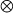International
Tables for
Crystallography
Volume B
Reciprocal space
Edited by U. Shmueli

International Tables for Crystallography (2006). Vol. B, ch. 1.3, p. 58   | 1 | 2 |

Section 1.3.3.3.3.3. The Johnson–Burrus family of algorithms

G. Bricognea

aMRC Laboratory of Molecular Biology, Hills Road, Cambridge CB2 2QH, England, and LURE, Bâtiment 209D, Université Paris-Sud, 91405 Orsay, France

1.3.3.3.3.3. The Johnson–Burrus family of algorithms

| top | pdf |

In order to explore systematically all possible algorithms for carrying out a given DFT computation, and to pick the one best suited to a given machine, attempts have been made to develop:

 (i) a high-level notation of describing all the ingredients of a DFT computation, including data permutation and data flow; (ii) a formal calculus capable of operating on these descriptions so as to represent all possible reorganizations of the computation; (iii) an automatic procedure for evaluating the performance of a given algorithm on a specific architecture.

Task (i)can be accomplished by systematic use of a tensor product notation to represent the various stages into which the DFT can be factored (reindexing, small transforms on subsets of indices, twiddle factors, digit-reversal permutations).

Task (ii)may for instance use the Winograd CBA normal form for each small transform, then apply the rules governing the rearrangement of tensor productand ordinary product × operations on matrices. The matching of these rearrangements to the architecture of a vector and/or parallel computer can be formalized algebraically [see e.g. Chapter 2 of Tolimieri et al. (1989)].

Task (iii)is a complex search which requires techniques such as dynamic programming (Bellman, 1958).

Johnson & Burrus (1983)have proposed and tested such a scheme to identify the optimal trade-offs between prime factor nesting and Winograd nesting of small Winograd transforms. In step (ii), they further decomposed the pre-addition matrix A and post-addition matrix C into several factors, so that the number of design options available becomes very large: the N-point DFT when N has four factors can be calculated in over 1012 distinct ways.

This large family of nested algorithms contains the prime factor algorithm and the Winograd algorithms as particular cases, but usually achieves greater efficiency than either by reducing the f.p. multiplication count while keeping the number of f.p. additions small.

There is little doubt that this systematic approach will be extended so as to incorporate all available methods of restructuring the DFT.

References

Bellman, R. (1958). Dynamic programming and stochastic control processes. Inf. Control, 1, 228–239.
Johnson, H. W. & Burrus, C. S. (1983). The design of optimal DFT algorithms using dynamic programming. IEEE Trans. Acoust. Speech Signal Process. 31, 378–387.
Tolimieri, R., An, M. & Lu, C. (1989). Algorithms for discrete Fourier transform and convolution. New York: Springer-Verlag.